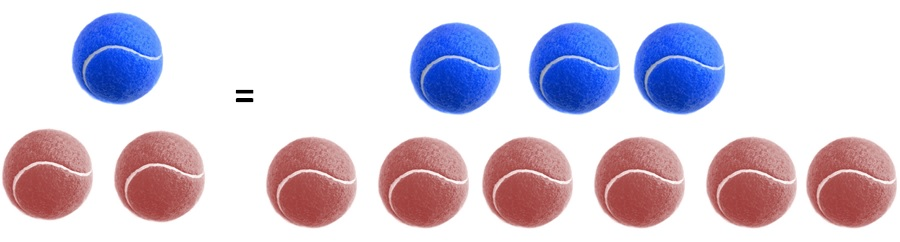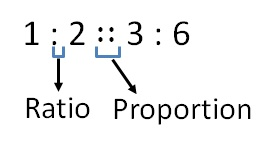Proportion

Chapter 12 Class 6 Ratio and Proportion
Concept wise

If two ratios are equal, we say they are in proportion

Example:

1/2 & 3/6∴ 1/2 = 3/6

i.e.

1 : 2 = 3 : 6

We also write it asAnd we say that,

1, 2, 3, 6 are in proportion

Note:

When we write 4 numbers in proportion

1 , 2, 3, 6

1 , 6 are called extreme terms

2 , 3 are called middle terms

#### Are 10, 20, 40, 80 in proportion?

 Ratio of 10 & 20 = 10/20 = 1/2   = 1 : 2 Ratio of 40 & 80 = 40/80 = 4/8 = 1/2 = 1 : 2

∴ 10 : 20 = 40 : 80

So, 10, 20, 40, 80 are in proportion

Learn in your speed, with individual attention - Teachoo Maths 1-on-1 Class

### Transcript

1 : 2 ∷ 3 : 6 Ratio Proportion